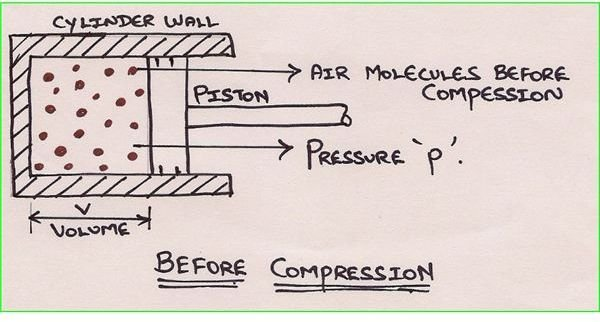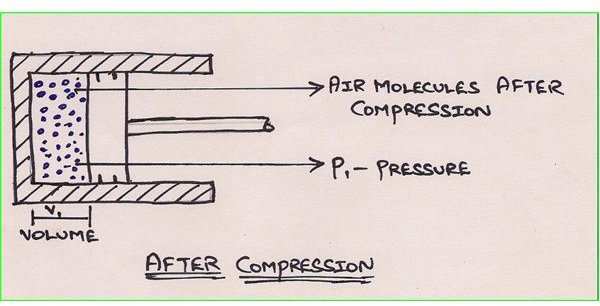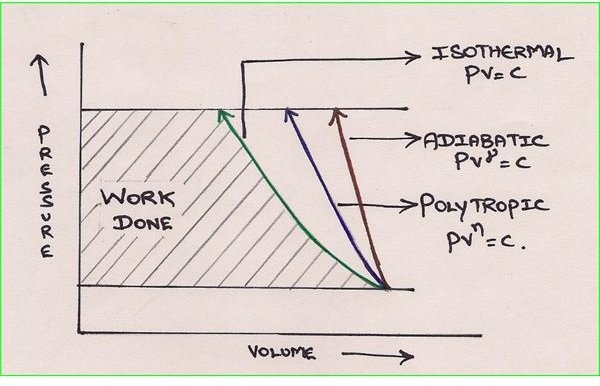# Air Compressor Theory: Air Compression Cycle & Formula

## Introduction:

The quantity of air available in our atmosphere is huge and every living organism require air (which has oxygen) to survive. Not only organisms, even machineries and engines require air for their proper and efficient operation. Thus air plays a vital role in industries and plants. But they are usually compressed so that energy is available to perform some work. In this article, let us understand the theory of compressing air and various associated laws.

## “Compressed air” What it means?

The free air has some density or the given mass of gas occupies certain volume in free space. The molecules of air occupy a certain volume in free space. By compressing, the molecules of air are made to come closer, by which they occupy less space when compared to earlier (when they were free). As the number of molecules of air increases in a given volume, the mass of air also increases. As the mass of air in the given volume increases, its density also increases. As the density increases, the pressure of air increases and thus becomes compressed air.## Basic Theory of Compressing Air:

The air we breathe has two major constituents. They are Nitrogen and Oxygen. The approximate composition of atmospheric air has 78% nitrogen, 21% oxygen and 1% other gases by volume and 76% nitrogen, 23% oxygen and 1% other gases by weight.

Even though air is not a “perfect” gas, the presence of nitrogen and oxygen in major proportion makes it obey very closely to a “perfect” or “ideal” gas. We all know that an “Ideal” gas obeys some laws. They are

1. Boyle’s law (PV=C).
2. Charles’s law (V/T = C).

The above two laws can be combined to form a combination law which can be represented as

PV/T = C.

## What happens to Air when it is compressed?

Keeping the combination law in mind, when the air is compressed, the pressure and temperature of the air increases as the volume of the space containing air reduces.

This can be understood better when used in Boyle’s law and Charles’s law separately.

Boyle’s Law:

PV=C. or P is inversely proportional to V.

As the volume of the space containing air reduces, the pressure increases.

Also when volume V, is kept constant, the pressure P, is directly proportional to the temperature T. Thus as the pressure of the air is increased due to compressing, the temperature also increases. Thus as a result of compressing air, the pressure and temperature increases as the volume decreases.

## Compression Cycles and Types:

Air can be compressed in two ways.

1. Isothermal compression.Isothermal compression:

The name of the process ISO is equal or same and thermal is heat or anything related to temperature. Thus isothermal compression is compressing air with no change in temperature or at constant temperature. But we have already discussed that as the pressure of air increases, the temperature also increases. So to achieve isothermal compression, the heat produced during compression has to be removed at the same rate to keep the temperature constant. Thus to achieve same rate of heat exchange, the design of compressor must be such that it should move slowly thus we have more time to extract the same amount of heat as we receive it. The cylinder bore must be small and require a high standard of cooling. Thus during isothermal compression, the work input is the least and involves no temperature change.

The isothermal compression process can be symbolically represented as PV = C.

Adiabatic or Isentropic Compression:

Adiabatic compression in simple terms means that no heat is given to or taken from the cylinder walls of the compressor. Also all work done in compressing the air is stored in the compressed air itself. Thus this process takes maximum energy input as no heat loss takes place through the cylinder walls. Thus theoretically, the compression process can be represented as PV^ γ = C.

## Actual or Polytropic Compression:

In the above two theoretical compression processes, there are huge practical constraints to achieve. Thus the actual compression process is termed as polytropic compression. From the curves shown in the figure, it is evident that the work done in adiabatic process is more than isothermal. So it is only isothermal compression which is desired in an air compressor. But in practice, isothermal compression is not possible to achieve. To achieve isothermal compression, if the piston movement in compressing air is slowed down and with small bore of the cylinder walls to extract the heat as received in the process of compression, then the desired air delivery rate would be a problem. This is the reason, compressors compress air polytropically. From the figure, it is evident that the polytropic curve is in between both extremes i.e., adiabatic and isothermal compression curves.

## Conclusion

In my next article, let us discuss about the actual air compression process and cycles involved followed by various parts of an air compressor.